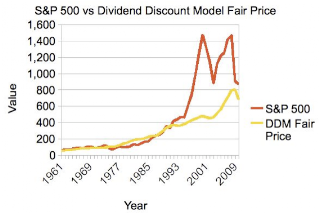## 2009-04-17

### S&P 500 Fair ValueEstimating the fair value of a stock is an exercise in predicting the future. Estimating the fair value of an entire index, such as the S&P 500 is not any easier. Luckily for us, it doesn't involve estimating the future of 500 companies simultaneously. We can treat the entire index as a single company (sort of like a conglomerate, with multiple divisions).

Many people like to predict future earnings and then apply a multiple (such as the P/E multiple) or forecast free cash flow year over year, apply a discount rate and add up the values. Others, like me, prefer to value a stock using the stream of dividends it will provide in the future, using the Dividend Discount Model (DDM).

Whatever method one uses, it boils down to three things:
1. Predicting future growth g (of earnings, cash flow, dividends)
2. Setting an appropriate discount rate r
3. Applying the appropriate model.
In this case, I will use:
1. A dividend growth rate inline with smoothed historical rates.
2. Use a discount rate that provides a reasonable historical fit, which a priori should be between 7 and 20% (another option is to use the risk-free rate of return, such as that of treasury bonds, but I don't like that option for various reasons that I won't discuss right now)
3. Use the Dividend Discount Model (DDM)
The DDM states that value = dividend / (discount rate r - growth rate g)

So, what is a good historical dividend growth rate? Let's look at the past to estimate that. The raw data can be found here.

Since 1960, the dividend rate has grown on average at 5.68% over the years.

The discount rate has varied a lot as investors took more or less risk depending on their perception at the time of risk, inflation and future growth. Fitting the DDM over the years to get a good approximation yields a 7.5% rate of discount (see the figure). This is the return rate an investor should demand for exposing his money to market risk. 7.5% seems appropriate as a long-term rate, even if it seems low right now, since times won't always be bad in the future, nor will they necessarily be good, like they were in the late 90's, when investors were demanding very little rates of return (even negative!).

Now, all we need is a starting point for the dividend the S&P500 index will pay an investor this year. Well, let's make an educated guess. In 2008, the dividend was \$28. Since then, many companies have cut their dividends, suspended them or gone bankrupt. Very few have raised their dividends. If we assume a 15% drop in dividends for 2009, the fair value of the S&P 500 should be 681, not the current 870.

See the figure above for a plot of the estimated fair value versus the real value since 1960 (2009 numbers are measured year-to-date and estimated are year-end).

Can it fall more? Of course. Can it go up more from here? Sure. The best bet is to buy companies that are undervalued based on the Dividend Discount Model and for which future prospects look solid.

As for the S&P 500, I wouldn't bet on a continued rally from here.